#Quality Digest

Featured Product
This Week in Quality Digest Live
Statistics Features
Donald J. Wheeler
Using process behavior charts in a clinical setting
Donald J. Wheeler
No one really understands kurtosis. Here’s why.
Alan Metzel
Introducing the Enhanced Perkin Tracker
Donald J. Wheeler
What you think you know may not be so
Tony Boobier
Why data leaders need to master words as well as statistics
Statistics News
New capability delivers deeper productivity insights to help manufacturers meet labor challenges
Day and a half workshop to learn, retain, and transfer GD&T knowledge across an organization
Elsmar Cove is a leading forum for quality and standards compliance
InfinityQS’ quality solutions have helped cold food and beverage manufacturers around the world optimize quality and safety
User friendly graphical user interface makes the R-based statistical engine easily accessible to anyone
Collect measurements, visual defect information, simple Go/No-Go situations from any online device
Good quality is adding an average of 11 percent to organizations’ revenue growth
Ability to subscribe with single-user minimum, floating license, and no long-term commitment
A guide for practitioners and managersBio

Statistics

## How to Convert ANSI/ASQ Z1.4 Sampling Plans Into Narrow-Limit Gauging Plans

### Reduce the amount of attribute inspection that must be done

Published: Wednesday, May 22, 2019 - 12:03

Anthony Chirico1 describes how narrow-limit gauging (NLG, aka compressed limit plans) can reduce enormously the required sample size, and therefore the inspection cost, of a traditional attribute sampling plan. The procedure consists of moving acceptance limits t standard deviations inside the engineering specifications, which increases the acceptable quality level (AQL) and therefore reduces the sample size necessary to detect an increase in the nonconforming fraction.

"Let's not turn our backs on MIL-STD-105, and let's start supporting its innovative application," adds Chirico. "The individuals who conceived and wrote this procedure were visionaries ahead of their time." Schilling, Ott, Mundel, Sommers, and the other authors cited by Chirico did not have access to the modern computing technology that makes NLG easily accessible to today's practitioners. Any spreadsheet program such as Excel can easily generate the operating characteristic (OC) curves for the original (ANSI/ASQ Z1.4) plan and corresponding compressed limit plan to ensure that they provide comparable protection against both the producer's risk of rejecting good lots and the consumer's risk of accepting bad ones. StatGraphics, Minitab, and similar packages can design acceptance sampling plans that are based on the binomial or hypergeometric distribution rather than the questionable (for high nonconforming fractions) Poisson approximation to the binomial distribution. The advantages of doing this, in terms of substantial reductions in mandatory but nonvalue inspection, are considerable.

### Prerequisites

Narrow-limit gauging is applicable when:
• Variables or real-number measurements can be checked on a go/no-go gauge.
• The critical-to-quality (CTQ) characteristic follows the normal distribution.
• Increases in the nonconforming fraction can be attributed exclusively to shifts in the process mean.

Almost any ANSI/ASQ Z1.4 (formerly MIL-STD 105) plan can be converted into an NLG plan with a much smaller sample size and an OC curve comparable to that of the original plan.

The assumption that the number of nonconforming items in a sample follows the Poisson approximation to the binomial distribution works best for large sample sizes and small nonconforming fractions. It is not reliable when the nonconforming fraction increases, as it must do in an NLG plan, but it is now easy to work around this issue. The documentation for StatGraphics 18 states that OC curve plans are designed such that the producer's risk is no greater than that specified (usually 5%) at the AQL, and the consumer's risk is no greater than that specified (usually 10%) at the rejectable quality level (RQL). The hypergeometric distribution is used to determine the chance of acceptance and, when the lot size far exceeds the sample size, we might as well be using the binomial distribution. The problematic reliance on the Poisson approximation therefore ceases to be an issue.

### How to define a compressed limit plan

Schilling2 gives the following approach for an NLG plan. The objective is to define tightened acceptance limits LSL + and/or USL ˗ tσ where
• LSL is the lower specification limit
• USL is the upper specification limit
• σ is the process standard deviation
t is the compression constant (in standard deviations)
This approach requires explicitly that the underlying process distribution be normal. Schilling adds that, when double specification limits are at least 6σ apart (that is, the process capability is 3 sigma), compressed limit plans can be applied to each specification limit. Remember that a process is not considered capable unless the process performance index is 4/3 (4 sigma) or greater. Another way of saying this is that we do not have to worry about the LSL and USL simultaneously.

Example: ANSI/ASQ Z1.4 gives, for code letter J, normal inspection, and AQL = 1 percent, sample size n = 80 and acceptance number c = 2. Define an NLG plan when the specification is tightened by t = 1 process standard deviation.

ANSI/ASQ Z1.4 plans do not have formal rejectable quality levels (RQLs), but it is common practice to pretend for analytical purposes, e.g., to define a zero acceptance number plan or sequential sampling plan, that the RQL is the nonconforming fraction for which the chance of acceptance (consumer's risk) is 10 percent. Table X-J of the standard gives a 95-percent chance of acceptance for p = 1.03 percent (so we will treat 1.03 percent rather than 1.00 percent as the AQL) and 10 percent for p = 6.52 percent. These calculations are based on the binomial distribution. When the X tables for operating characteristic curves (single sampling plans) rely on the Poisson distribution instead, we can still get the binomial-related AQL and RQL from the relationship between the binomial distribution and the F distribution (equation 1).

Equation 1: p as a function of n, c, and acceptance probability Pawhere F is the Pa quantile (left tail area) of the F distribution with 2(n ˗ c) numerator degrees of freedom, and 2(c + 1) denominator degrees of freedom.

In this example, where we want to set the AQL and RQL at the 95 percent and 10 percent chances of acceptance (chances of getting two or fewer nonconforming items) respectively,This is done easily in Excel using F.INV(Pa,2(n ˗ c),2(c + 1)) where F.INV returns the quantile, i.e., the left tail, of the F distribution for the indicated chance of getting c or fewer out of n nonconformances. Pa, n, and c refer to the cells that contain the acceptance probability (cumulative binomial probability from 0 to c), sample size, and acceptance number.

(A spreadsheet is available that contains the calculations for the examples and also the reproduction of Table 2 from the Cameron reference.)

For a single specification limit, the approach prescribed by Schilling is as follows.

Step 1. Determine the standard normal deviate for the producer's quality level (acceptable quality level, AQL) and consumer's quality level (rejectable quality level, RQL) as shown in equation set 2.

Equation set 2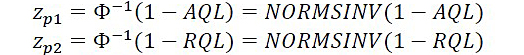where p1 is the AQL and p2 the RQL when the gauge is set at the specification limit. Φ˗1 is the inverse cumulative normal distribution function, and it returns the standard normal deviate of its argument.

This is easily deployable in Excel where AQL and RQL are the cells that hold these values or are named variables. In this case, zp1 = 2.315 and zp2 = 1.513. This means the nonconforming fraction equals the AQL when the process mean is 2.315 standard deviations from the specification limit, and the RQL when the process mean is 1.513 standard deviations from the specification limit (figure 1, Minitab graphs).Figure 1: zp1 and zp2

Step 2. Compute zg1 = zp1 ˗ t and zg2 = zp2 ˗ t. These are the number of standard deviations between the mean of the lot under inspection and the compressed limits for which the nonconforming fractions will correspond to the original plan's AQL and RQL respectively. In this case, zg1 = 2.315 ˗ 1 = 1.315 and zg2 = 1.513 ˗ 1 = 0.513.

Step 3. Use equation set 3 to compute the tail areas where pg1 and pg2 are the expected nonconforming fractions at the compressed limits for the AQL and RQL respectively. Another way of saying this is that pg1 and pg2 are the AQL and RQL of the compressed limit plan for which the producer's and consumer's risks should be 0.05 and 0.10 respectively.

Equation set 3where pg1 is the fraction of parts outside the compressed limit when the original nonconforming fraction p equals the original AQL, and pg2 the fraction of parts outside the compressed limit when the original nonconforming fraction p equals the original RQL. Φ is the cumulative standard normal distribution. In this case,Figure 2 (Minitab graphs) illustrates the fraction of parts outside the compressed limits.Figure 2: pg1 and pg2

In other words, when the actual nonconforming fraction is 0.0103, the fraction of parts outside the compressed limit will be 0.0943 and, when the actual nonconforming fraction is 0.0642, the fraction outside the compressed limit will be 0.304. Equation 4 gives the general relationship between the nonconforming fraction p when the gauge is set at the specification limit, and the fraction pg when the acceptance limit is tightened by t process standard deviations

Equation 4where Φ˗1(1 ˗ p) is the standard normal deviate for (1 ˗ p), and this is illustrated in figure 3 (Minitab scatter plot). p and t refer again to the relevant cells in Excel for the calculation shown at right. This relationship will be useful for comparing the OC curves for the original and compressed limit plans.Figure 3: Correspondence between nonconforming fraction (p) and fraction beyond compressed limit (pg)

Step 4. Compute the operating ratio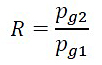which is in this case 0.304/0.0943 = 3.367.

Step 5. Use the table of unity factors (T5˗1 in Schilling2, and it also appears in Cameron3) to get an acceptance sampling plan with sample size n, acceptance number c, producer's risk α, and consumer's risk ß that matches the given operating ratio.

Cameron3 says to select the larger of the tabulated R values that corresponds to that calculated in step 4. Cameron uses an example in which R = 2.6. The tabulated values are 2.497 for c = 10 and 2.619 for c = 9, so the row for c = 9 is used. In this case, the tabulated values are 3.206 for c = 6 and 3.509 for c = 5, so use the row for c = 5 for which np1 is 2.613. 2.613 ÷ 0.094 = 27.8 which rounds up to 28. We are currently relying on the Poisson approximation. (StatGraphics gets n = 29, c = 5 from the hypergeometric distribution.)

The next step is to show that the compressed limit plan offers protection against poor quality that is comparable to that of the original plan. Figure 4 (StatGraphics multiple scatter plot) compares the OC curves for the two plans along with pg as a function of p. The points for the graph are easily developed in Excel as follows. Create four columns:
1. p for the original nonconforming fraction, e.g., in increments of 0.001, 0.002, or whatever is appropriate.
2. Corresponding fraction outside the compressed limit pg = NORMSDIST(NORMSINV(p)+t) noting that NORMSINV(p) will be negative for p<0.50.
3. Probability of acceptance given nonconforming fraction p, ANSI/ASQ Z1.4 sample size n and acceptance number c: Pa(p) =BINOM.DIST(c,n,p,1) where p is the corresponding cell in the first column, and c and n are in fixed cells or are named variables. The "1" as the last argument specifies the cumulative rather than the point distribution.
4. Probability of acceptance Pa(pg) given pg = BINOM.DIST(c_compressed,n_compressed,pg,1) where c_compressed and n_compressed are the acceptance number and sample size for the narrow limit gauging plan.

We can then plot pg, Pa(p), and Pa(pg) vs. p to show (hopefully) that the two operating characteristic curves are essentially identical, or at least that the one for the NLG plan provides superior protection against poor quality at the cost of rejecting more lots at the AQL. We cannot disregard the latter issue because rejection of a lot will invoke ANSI/ASQ Z1.4's switching rules.

Figure 4 (StatGraphics plot of data from Excel) shows the two OC curves. The set of points whose values increase from left to right are the fractions (pg) that exceed the compressed limits as a function of the nonconforming fraction for the original specification limit. The set of points whose values decrease from left to right are the chances of acceptance (c or fewer items found outside the limit) for the original (Pa(p)) and compressed limit (Pa(p_g) sampling plans. They are almost indistinguishable.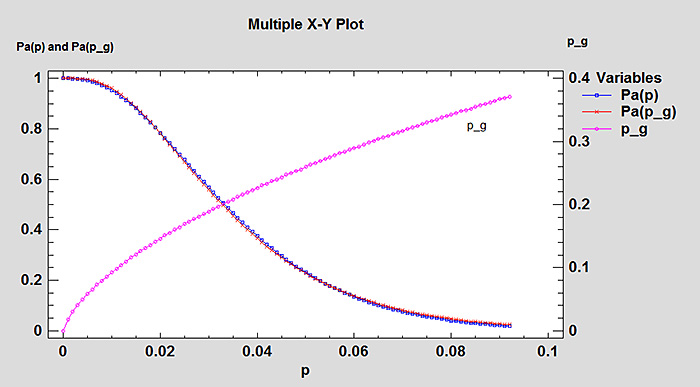Figure 4: OC curve of initial and compressed limit plans

The underlying calculations can be performed in a couple of minutes in Excel (once the four-column table is set up), and the results graphed in Excel although StatGraphics and Minitab produce somewhat better looking graphs. This technology was not available to the originators of narrow-limit gauging, and it should go a long way toward making their methods easily accessible to modern practitioners. If we want our internal or external customer to accept an NLG plan, it is very helpful to show the customer that the OC curve is almost identical to that of the original sampling plan.

### Optimal narrow-limit plan

Schilling2 describes how nomographs can be used to obtain an optimum narrow-limit plan, which can reduce the sample size by about 80 percent of the difference between the attribute (ANSI/ASQ Z1.4) plan and corresponding variables (ANSI/ASQ Z1.9) plan where the standard deviation is known (Section D of the standard). A heuristic approach (Schilling and Sommers4) uses the ANSI/ASQ Z1.9 plan as shown in equation set 5.

Equation set 5
n = 1.5nv where nv is the sample size for the variables plan
t = k where k is the acceptance constant for the variables plan
c = 0.75nv ˗ 0.67

For sample code letter J and AQL = 1 percent, nv = 12 and k = 1.88. Then the sample size for the compressed limit plan is 18, t = 1.88, and the acceptance number is 8.33. When c = 8, the chance of acceptance is somewhat less than for the original ANSI/ASQ Z1.4 plan and, when c = 9, it is considerably more so we would have to use c = 8. This results in a substantially higher risk of rejecting lots at the AQL. Noting that rejections will invoke the plan's switching rules, we must ask whether the reduction in sample size from 28 to 18 is worth this disadvantage.

Table T13-5 from Schilling defines n = 20, t = 1.93, and c = 10 as the optimal narrow limit plan for this situation in which the compressed limit plan and original plan have roughly equivalent OC curves (figure 5, StatGraphics). The optimal narrow limit plan has, however, a slightly higher chance of accepting the sample when the nonconforming fraction exceeds the RQL.Figure 5: p_g and OC curve for n = 80, c = 2, and n = 20, c = 10, t = 1.93

Table T13-5 from Schilling, and also Schilling and Sommers4, tabulate optimal compressed limit plans for a wide array of ANSI/ASQ Z1.4 plans. It is recommended, however, to generate the OC curves for both the ANSI/ASQ Z1.4 plan and the compressed limit plan to ensure that they are at least roughly identical, and especially that the compressed limit plan offers at least equal protection against poor quality.

### Massive sample size reductions

The plan for code letter Q, AQL = 0.01 percent, which normally has a sample size of 1,250 and acceptance number 0, can be deployed (per Table T13-5 from Schilling) as a compressed limit plan with a sample size of only 12, t = 3.46, and acceptance number 6. This is also what I get when I let StatGraphics design the plan based on pg1 and pg2. There is however a considerable mismatch between the OC curves, with the compressed limit plan having a somewhat higher chance of rejecting lots when the nonconforming fraction is below the RQL. Noting that, if AQL = 0.01 percent, the process must be highly capable so there should be no problem with trying t = 2 (which is much less than 3.46).

The resulting plan has n = 14, c = 1 but there is a substantial mismatch between the OC curves—quite likely because R = 6.96 which is much closer to the tabulated entry for c = 2 than c = 1. StatGraphics designs the plan as n = 28, c = 2, while use of the table of unity factors for c = 2 delivers n = 32. We might expect the Poisson approximation to work quite well for a sample size of 1,250 when the nonconforming fraction is far less than 1 percent, but the compressed limit plan involves much smaller samples and much larger fractions outside the compressed limits.

Figure 6 (StatGraphics) shows clearly that it is better to allow StatGraphics or Minitab to use the hypergeometric or binomial distribution to design the plan rather than relying on the Poisson approximation. The important takeaway here is that the required sample is now 28 rather than 1,250, which can result in enormous inspection cost savings if the go/no-go gauge is manually operated rather than automated.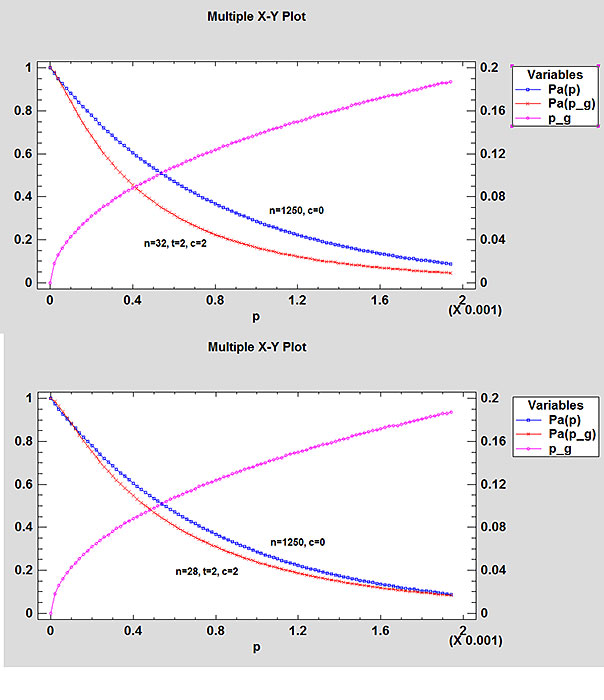Figure 6: Compressed limit plans (t = 2) for code letter Q, AQL = 0.01 percent

### What happens for smaller sample sizes

The Poisson approximation to the binomial distribution works best for large sample sizes and small probabilities of occurrence. Try sample code letter F with AQL = 6.5 percent for which n = 20 and c = 3, and generate a compressed limit plan with t = 1. We don't expect the Poisson distribution to work very well for the original sample plan, let alone the compressed one.

Table X-F gives the relevant points on the OC curve as (0.0713,0.95) and (0.304,0.10) for the AQL and RQL at which the respective chances of acceptance are 95 percent and 10 percent. AQL = 0.0714 (from the F distribution as shown previously) actually works a little better. Then:
zp1 = 1.465 and zp2 = 0.512
zg1 = 0.465 and zg2 = -0.488
pg1 = 0.321 and pg2 = 0.687
R = 2.142

From table 2 in Cameron3, c = 10 and np1 = 6.169.

This gives us a compressed limit plan with n = 19.23 (round up to 20) and c = 10, which doesn't do anything for us in terms of the sample size. The OC curves actually match relatively well as shown by figure 7, with the compressed limit plan having a slightly better chance of acceptance for good quality lots and a somewhat worse chance of acceptance for poor quality lots (figure 7).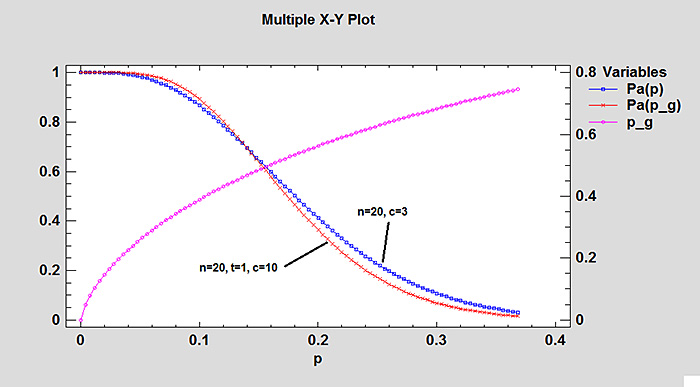Figure 7: OC curves for n = 20, c = 3, and n = 20, t = 1, c = 10

If however, we let StatGraphics define the compressed limit plan for AQL = 32.1 percent with producer's risk 5 percent, and RQL = 68.7 percent with consumer's risk 10 percent, we get n = 16 and c = 8 for which the OC curves have a much better match as shown in figure 8.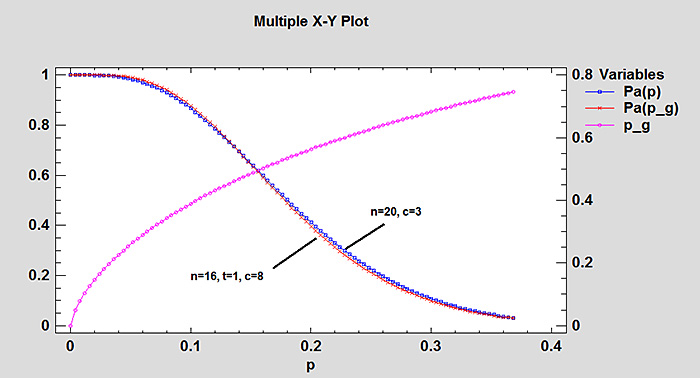Figure 8: OC curves for n = 20, c = 3, and n = 16, t = 1, c = 8

This still doesn't save us much in the way of sampling, but the takeaway is that allowing StatGraphics (or Minitab, which has the same capability and gets the same results for this example) to design the sampling plan without relying on the Poisson approximation overcomes the drawback of needing to rely on it.

### Reproduction of table 2 from Cameron3

The procedure in Cameron3 assumes the number of nonconformances follows a Poisson rather than a binomial distribution, an assumption that becomes questionable as the nonconforming fraction increases (because the Poisson approximation works best for large samples with low probabilities of occurrence). Cameron3 computes R as follows where α is the producer's risk and ß the consumer's risk, which are customarily set at 0.05 and 0.10, respectively.where the chi square values are for the right tail, i.e., one minus the cumulative distribution.

As an example, for α = 0.05, ß = 0.10, and c = 3,and then R = 4.889 (4.890) which matches the tabulated value.

This is easily reproduced in Excel with

=CHISQ.INV.RT(C\$4,2*\$A10+2)/CHISQ.INV.RT(1-C\$3,2*\$A10+2)
where C\$4 = ß, C\$3 = α, and \$A10 = c. The resulting Excel table (table 1) matches the one in the reference.Table 1: Unity factors for a = 0.05

n × p1 (the Poisson mean) that will give a 1 ˗ α chance of getting c or fewer defects is meanwhileAs an example, for c = 3 and a = 0.05,half of which is 1.367. This is =0.5*CHISQ.INV.RT(1-\$B\$3,2*A10+2) in Excel.

The table can be developed further for producer's risks other than 0.05, but the one most likely to be used sets the producer's risk at 0.05 and the consumer's risk at 0.10. Again, however, it is probably better to use the hypergeometric or binomial distribution to define the sampling plan to avoid any issues related to the Poisson approximation.

### Conclusion

Narrow-limit gauging offers a substantial reduction to the amount of attribute inspection that must be done under ANSI/ASQ Z1.4. The reduction can be enormous at lower AQLs, where the ANSI/ASQ Z1.4 plan could require samples exceeding 1,000. Use of this procedure is, however, subject to the following conditions:
1. The critical-to-quality characteristic must follow the normal distribution.
2. The nonconforming fraction must be a function of shifts in the process mean. If the process variation changes instead, the underlying assumptions are no longer valid.
3. The process must be capable when two-sided specification limits are involved.

Always generate the OC curves for the original ANSI/ASQ Z1.4 plan and the compressed limit plan to ensure that they are at least similar, and that the compressed limit plan does not create unacceptable producer's or consumer's risks. Remember that rejection of good lots can tighten the inspection plan according to the switching rules, while of course acceptance of bad lots exposes the customer to poor quality. It is also probably better to let a software package like StatGraphics or Minitab, and there are doubtlessly others with similar capability, to define the compressed limit plan while using the hypergeometric or binomial distribution rather than relying on the Poisson approximation.

A spreadsheet is available that contains the calculations for the examples and also the reproduction of Table 2 from the Cameron reference.

References
1. Chirico, Anthony. 2018. "Applying the Procedures of MIL-STD-105 to Imaginary Limits." Quality Digest, October 15 2018.
2. Schilling, Edward. 1982.  Acceptance Sampling in Quality Control. New York: Marcel Dekker
3. Cameron J.M., (1952). "Tables for Constructing and Computing the Operating Characteristics of Single Sampling Plans," Industrial Quality Control, Vol.9, No.1, pp. 37–39. http://asq.org/qic/display-item/index.html?item=3953 for ASQ members
4. Schilling, E.G. and Sommers, D.J. 1981. "Two-Point Optimal Narrow Limit Plans with Applications to MIL-STD-105D," Journal of Quality Technology, 13(2), 83–92 http://asq.org/qic/display-item/index.html?item=5383 access currently free to ASQ members.

### Discuss